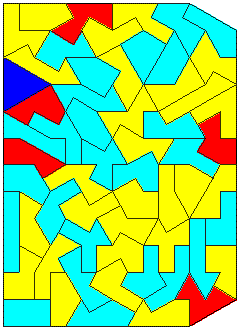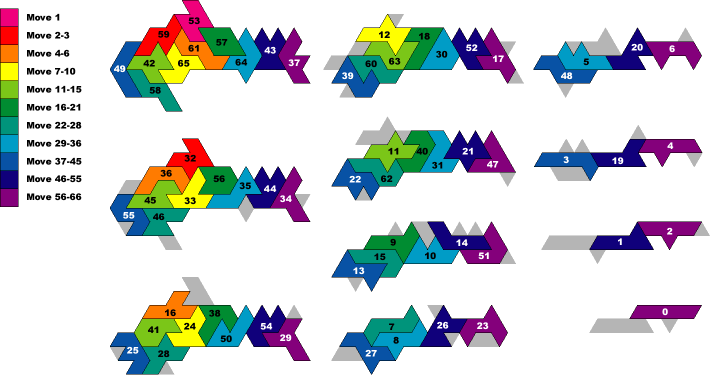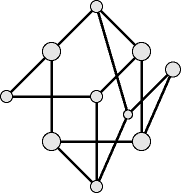OPEN CONTESTS PAGE
If you're willing to risk \$50 or more on a problem, write me.
You don't have to pay *me* anything - just pay whoever solves your problem!

Prize Problem -- \$500. Claim: For an integer n > 2, there are no solutions in the Gaussian integers to the equation xn+yn=zn. I hereby offer \$500 for a counterexample, with requirements |x y z| > 0 and {x,y,z}Gaussian Integers. (I have no interest in impossibility proofs). Dave Rusin pointed me to a math-atlas note.

There are many more such prize problems on my Neglected Gaussians page.

Prize Problem -- \$100.  Theoretically, squares of size 1-47 could cover a 188x190 rectangle. Squares of size 1-48 might cover a 194x196 rectangle.  For \$100, find a perfect rectangle made from sequentially sized squares.

Prize Problem -- \$100.  Dave Greene offers a \$100 prize to anyone that can build a small stable glider reflector. (26 April 2013 -- Found by Mike Playle)

Prize Puzzle.  A \$1.5 million prize for the first program that can play Go at a professional level.  This is the the Ing Chang-Ki Prize.

Prize Puzzle.  A \$1.0 million prize to the first one to prove or disprove the Riemann Hypothesis. The Clay Mathematics Institute of Cambridge, Massachusetts (CMI) has named seven “Millennium Prize Problems.”  The Board of Directors of CMI have designated a \$7 million prize fund for the solution to these problems, with \$1 million allocated to each.  You can see more details at www.claymath.org.  The full list of problems: P versus NP, The Hodge Conjecture, The Poincaré Conjecture, The Reimann Hypothesis, Yang-Mills Existence and Mass Gap, Navier-Stokes Existence and Smoothness, and The Birch and Swinnerton-Dyer Conjecture. On the Riemann Hypothesis, the first hundred billion zeroes have been calculated by Zetagrid, without any counterexamples so far.|z(1/2 + b I)| for b = 0 to 85.  Note how the function always goes to zero.

Prize puzzle.  A \$100,000 prize for the first verified prime number with more than 10,000,000 digits.  See eff.org for more details.  www.mersenne.org is the main solving organization.  233219313 - 1 would be the first number to check. The smallest prime factor of 10^10000000! + 1 would also work.

Prize puzzle.  A \$100,000 prize for the first to solve The Beal Conjecture.  If Ax +By = Cz , where A, B, C, x, y and z are positive integers and x, y and z are all greater than 2, then A, B and C must have a common factor.

Prize puzzle. ZetaGrid is offering the following four prizes (see http://www.zetagrid.net/zeta/prizes.html for more rules):
1. \$10 (USD) will be awarded to the first person who discovers the first two zeros which have a distance less than 10^-6, using the software provided by ZetaGrid.
2. \$100 (USD) will be awarded to the first person who discovers the first two zeros which have a distance less than 10^-7, using the software provided by ZetaGrid.
3. \$1,000 (USD) will be awarded to the first person who discovers a nontrivial zero which is not on the critical line using the software
provided by ZetaGrid and before the Riemann Hypothesis disproved.
4. Up to \$1,000,000 (USD) award to the first 100 top producers of ZetaGrid if Sebastian Wedeniwski wins the \$1 million prize for a proof of the Riemann Hypothesis from Clay Mathematics Institute by using the results or the statistical summaries of ZetaGrid.

Prize puzzle.  A \$100 prize is offered by Martin Gardner's for the first to make a 3x3 magic square where all entries are different perfect squares.  Or prove that this is impossible.  The best known construction is below, by Lee Sallows.  One diagonal fails to add up to the magic constant of 147^2.  Adam Dewbery (and many others) proved that the central square of a solution must have several properties (I've added to it).  Interesting things like 472+792 = 232+892 = 132+912 = 352+752 = 652+652 pop up during analysis.  Near-magic squares are completely characterized (near-magic squares are ones with all rows, all columns, and one of the two diagonals adding to the magic constant).  For any congruent number  n  (an integer that is the area of some rational right triangle), find 3 points on the elliptic curve y2 = x3-nx, such that each point is the double of another point on the curve.  Each point corresponds to a 3-term arithmetic progression.  If these three progressions are distinct, then they generate a near-magic square. See John Robertson's paper Magic Squares of Squares in the October 1996 Mathematics Magazine, pp. 289 ff.   This problem is now famous enough that the only proofs I will consider are those published in refereed mathematical journal.

 1272 462 582 22 1132 942 742 822 972
Lee Sallows' Almost-Perfect Square Square.  From CFF 45.

Several prize problems are at http://cedar.evansville.edu/~ck6/integer/unsolved.html.

Prize Puzzle. \$50 for a complete solution. The Logistic Map, x -> a x(1-x). At seanet.com, exact answers are given for cases a=2 and a=4. The first one -- after n iterations of x -> 2 x(1-x), the value of x will be (1 - Exp[2^n Log[1 - 2 x]])/2 . As a tough problem (I don't have an answer), what is the exact formula for x -> 3 x(1-x) after n iterations? If you can solve this, or if you know of existing literature on related exact formulas, please write me. The below piece of code shows the standard diagram, with the first hundred iterations tossed out. You can see iteractive versions of the logistic map at Wolfram.com,Geometry Center, Gallery of Mathematics, University of Tokyo, Burning Chaos Page, Chaos and Order, and Mathpost. It's related to the Lyapunov Exponent. Nice explanations of this are given by J C Sprott, and the Chaos Hypertextbook.

````Prize Puzzle.  A \$100.00 prize will go to the first solver of Livio Zucca's Big Pizza Puzzle - whether you solve the puzzle or prove it impossible.Livio Zucca's Big Pizza Puzzle -- False Solution

Prize Puzzle.  How many pieces are necessary to make the 97 two tile combinations of Chaos Tiles simultaneously?  \$50 to the first person that can do it with 50 Veks and 40 Kays, or fewer. (No-one entered the original contest, which expired 7 Dec 2000)There is no better prize for solving a mathematical puzzle than a puzzle itself. Create unique one of a kind puzzles with your favorite algorithm, veks or design.SOLVED PUZZLES
Prize Puzzle.  A \$1.6 million prize to the first solver of the Eternity Puzzle.Solved by Guenter Stertenbrink.

Prize Puzzle.  \$100 dollars.. Solved by William Watson and Timothy Firman.   See solution here.Prize Puzzle\$100 dollars.  Solved by Patrick Hamlyn.  Put the 59 hexadudes together to make a convex shape.Prize Puzzle\$100 dollars.  Solved by Patrick Hamlyn.  Arrange the 66 octiamonds to make 11 identical shapes of 6 pieces.Prize Puzzle.  A \$50 prize will go to the first to solve or prove impossible Octiamond SolitaireSolved by Wei-Hwa Huang
At every stage of the game, each piece must be completely supported.  Use the full set of 66 octiamonds, without reusing any.
Start:  Put one octiamond piece down.  This is level one.
Stage 2:  Add an octiamond to level one.  Put another octiamond on top, starting level two.
Stage 3:  Add an octiamond to level one, then add another to level two, then put another on top to make level three.
...
Stage 11:  Add an octiamond to level one, then add another to level two, then add another on level three, ..., then put the last octiamond on top to make level eleven."The algorithm used to solve it worked from the top of the "pyramid" and searched for possible moves downwards.  Retrograde analysis, in a sense.  Note that the longer pieces are at the top, while the compact, squat pieces are at the bottom."

Petersen Graph Zome Challenge -- \$100.  My Petersen Graph Zome challenge has been solved by David MacMahon. It's not possible. If you have a plug-in like Cosmo, you can examine his VRML constructions. His concept is described at his Analytic page. He put the solving technique on his Petersen page. The Problem: Arrange 10 nodes and 15 blue struts of the same length to make the Petersen Graph (above).  No struts may touch.  Here is a 14 strut construct by Wei-Hwa Huang.  I've also found several 14 strut constructions.  A 15 strut construction may not be possible.  A computer proof of impossibility is also acceptable.